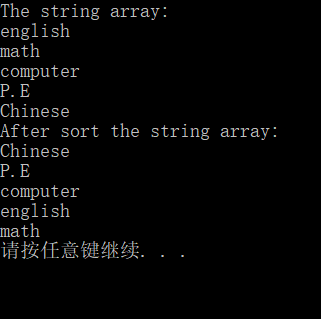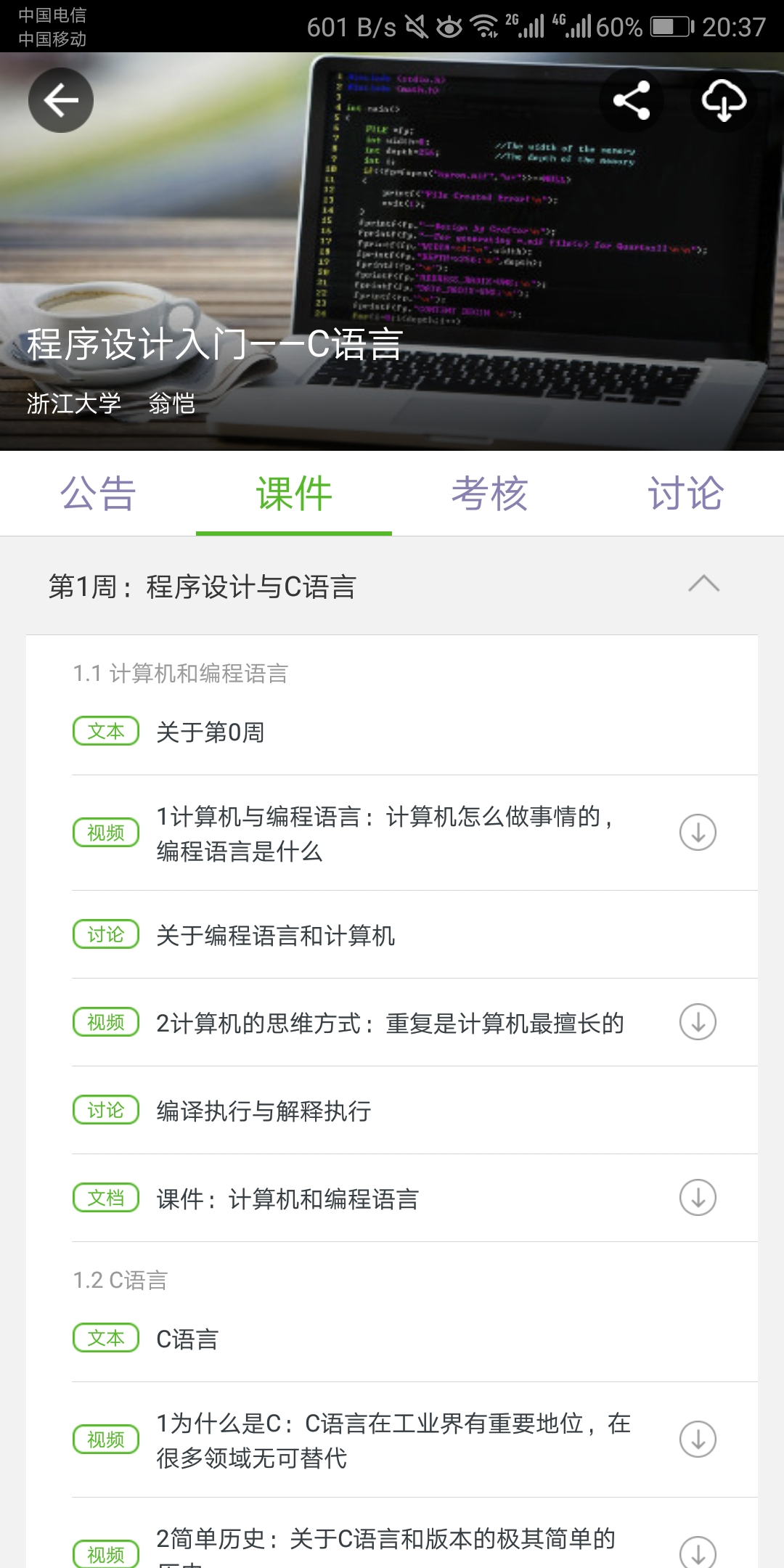# 第二次博客作业

1：

#include<stdio.h>
#include<stdlib.h>
main()
{
char a,ch,*p;
p=a;
int count=0,i;

gets(a);

ch=getchar();
for(;*p!='\0';*p++)
{
if(*p==ch)
count++;
else continue;
}
printf("%d\n",count);
system("pause");
}2：

#include <stdio.h>1
#include <stdlib.h>
#include <string.h>
void selectsort(char a[],int n)
{     int i,z,j;
char x;
for(i=0;i<n;i++)
{
z=i;
for(j=i+1;j<n;j++)
if(strcmp(a[j],a[z]) < 0)
z=j;
if(z>i)
{
strcpy(x,a[i]);
strcpy(a[i],a[z]);
strcpy(a[z],x);
}     }}
int main()
{
int i;    char str={"english","math","computer","P.E","Chinese"};
printf("The string array:\n");
for(i = 0;i < 5;i++)
printf("%s\n",str[i]);
selectsort(str,5);
printf("After sort the string array:\n");
for(i = 0;i < 5;i++)
printf("%s\n",str[i]);
system("PAUSE");
return 0;
}四：posted @ 2018-04-07 20:45  汪志恒  阅读(133)  评论(0编辑  收藏  举报